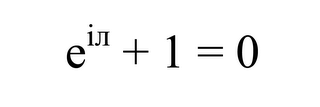Tuesday, August 22, 2006

EULER'S IDENTITYI don't completely understand this one, other than the fact that it contains imaginary pie. I understand the formula.. but can a mathematician explain the slogan?

At 7:20 AM,Ashwin said...

So... I am a mathematician and here is the (brief) explanation. The Real number line is called R. The 2-D plane is R x R. Any point (x,y) on this plane is described in vector form as x*x_hat + y*y_hat where x_hat and y_hat are unit vectors along the two axis. We can instead give this real plane a complex structure by considering the x-axis as a Real axis and the y-axis as the Imaginary axis. Now any point (x,y) has a vector description of x + y*i (the real unit vector is not written, the complex unit vector is i). This is saying that we go x units along the real axis then turn in the i direction (90 degrees) and go y units. The resulting vector points from the origin (0,0) to the point (x,y). With me so far ? So now we have created a new number... a "Complex number." The Complex plane C "looks like" R x R and every complex number has a real part and an imaginary part. The cool thing is that the set of real numbers is just the set of complex numbers with 0 imaginary part, i.e. numbers in the complex plane that look like (x,0). Another cool thing is that using basic trignometry we can describe any complex number x + y*i as r*exp( i*theta ) where r = sqrt(x^2+y^2) (by pythagorus theorem) and theta = arctan(y/x). I don't want to go into more of the trig derivation so just take it on faith for now. More intuitively think about how an ant gets to get to the point (x,y) on the plane .... it walks x units along the real axis, turns 90 degrees and walks y units in the complex direction. Alternatively, it could walk r units along the x axis (where r is the distance from (0,0) to (x,y)) and rotate an angle theta. So if the ant wanted to get to (1,0) it would walk r=1 and rotate theta=0, i.e. 1+0*i = 1*exp(i*0). To get to (0,1) it walks r=1 and rotates 90 degrees ( Pi/2 radians ), i.e. 0 + 1*i = 1*exp(i*Pi/2). To get to (-1,0) it walks r=1 and rotates 180 degrees ( Pi radians ), i.e. -1 + 0*i = 1 * exp(i*Pi) which is Euler's Identity ..... -1 = exp(i*Pi)

At 7:23 AM,Ashwin said...

crap .. just realized that you didn't want an explanation of the formula but the slogan. will look for that

At 7:26 AM,Ashwin said...

Check this out. http://en.wikipedia.org/wiki/Euler's_identity

At 6:31 PM,Anonymous said...

After proving the identity in a lecture, Benjamin Peirce, a noted nineteenth century mathematician and Harvard professor, said, "It is absolutely paradoxical; we cannot understand it, and we don't know what it means, but we have proved it, and therefore we know it must be the truth." 

Stanford mathematics professor Keith Devlin says, "Like a Shakespearean sonnet that captures the very essence of love, or a painting that brings out the beauty of the human form that is far more than just skin deep, Euler's equation reaches down into the very depths of existence."

At 12:34 PM,Steve said...

wow... explanation fail

At 10:30 AM,Anonymous said...

How about "as knowledge grows, life approaches a limit of -1?

At 3:52 PM,Anonymous said...

Crescat scientia vita = -1